Difference between revisions of "Jacobson ring"

This article defines a property of commutative unital rings; a property that can be evaluated for a commutative unital ring
View all properties of commutative unital rings
VIEW RELATED: Commutative unital ring property implications | Commutative unital ring property non-implications |Commutative unital ring metaproperty satisfactions | Commutative unital ring metaproperty dissatisfactions | Commutative unital ring property satisfactions | Commutative unital ring property dissatisfactions
This property of commutative unital rings is completely determined by the spectrum, viewed as an abstract topological space. The corresponding property of topological spaces is: set of closed points in any closed subset is dense

View other properties of commutative unital rings determined by the spectrum

History

Origin of the term

The term Jacobson ring was used by Krull in honour of Jacobson, who studied intersections of maximal ideals.

Alternative terminology

The term Hilbert ring or Hilbertian ring is also used because such rings are closely related to the Hilbert nullstellensatz.

Definition

Symbol-free definition

The following are equivalent definitions of Jacobson ring.

No. Shorthand A commutative unital ring is termed a Jacobson ring or Hilbert ring if ... A commutative unital ring$R$ is termed a Jacobson ring or Hilbert ring if ...
1 prime as intersection of maximals every prime ideal in it is an intersection of maximal ideals. for every prime ideal$P$ of$R$,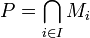$P = \bigcap_{i \in I} M_i$ where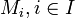$M_i, i \in I$ is the set of all maximal ideals of$R$ containing$P$.
2 radical as intersection of maximals every radical ideal in it is an intersection of maximal ideals for every radical ideal$J$ of$R$,$J = \bigcap_{i \in I} M_i$ where$M_i, i \in I$ is the set of all maximal ideals of$R$ containing$J$.
3 spectrum: closed points dense in the spectrum, the set of closed points in any closed set is dense in the spectrum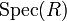$\operatorname{Spec}(R)$, any closed subset$C$ has the property that the set of closed points in$C$ is a dense subset of$C$.
4 quotient: nilradical equals Jacobson ring for every quotient ring, the nilradical equals the Jacobson radical for every ideal$I$, the quotient ring$R/I$ has the property that the nilradical of$R/I$ (i.e., the set of nilpotent elements, or equivalently, the intersection of all prime ideals) equals the Jacobson radical (the set of elements such that 1 + any multiple of the element is invertible, or equivalently, the intersection of all maximal ideals).
5 localization a field implies a field for any prime ideal, if the quotient contains an element at which its localization is a field, then the quotient is itself a field. for any prime ideal$P$ and any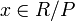$x \in R/P$, consider the localization of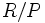$R/P$ at the set of powers of$x$. If the localization is a field, then$R/P$ must itself be a field.

Equivalence of definitions

The equivalence of the first three definition follows from the definitions of the terms involved. In particular, it uses the fact that in any commutative unital ring, any radical ideal is an intersection of prime ideals.

The equivalence with the fourth condition is termed Rabinowitch's trick.

Metaproperties

Metaproperty name Satisfied? Proof Statement with symbols
polynomial-closed property of commutative unital rings Yes Jacobson is polynomial-closed Suppose$R$ is a Jacobson ring. Then, the polynomial ring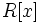$R[x]$ is also a Jacobson ring.
quotient-closed property of commutative unital rings Yes Jacobson is quotient-closed If$R$ is a Jacobson ring and$I$ is an ideal in$R$, then the quotient ring$R/I$ is also a Jacobson ring.

Relation with other properties

Stronger properties

Property Meaning Proof of implication Proof of strictness (reverse implication failure) Intermediate notions
polynomial ring over a field polynomial ring of the form$K[x]$ where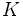$K$ is a field. Template:Intermeidate notions short
multivariate polynomial ring over a field of the form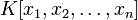$K[x_1,x_2,\dots,x_n]$ where$K$ is a field. click here

Opposite properties

A local domain that is not a field is not Jacobson. More generally, any local ring that has prime ideals other than the maximal ideal is not Jacobson.

Metaproperties

Closure under taking quotient rings

This property of commutative unital rings is quotient-closed: the quotient ring of any ring with this property, by any ideal in it, also has this property

View other quotient-closed properties of commutative unital rings

A quotient of a Jacobson ring by any ideal is also a Jacobson ring. This is clear, for instance, if we use the characterization in terms of every quotient ring having equal nilradical and Jacobson radical.For full proof, refer: Jacobson is quotient-closed

Closure under taking the polynomial ring

This property of commutative unital rings is polynomial-closed: it is closed under the operation of taking the polynomial ring. In other words, if$R$ is a commutative unital ring satisfying the property, so is$R[x]$

View other polynomial-closed properties of commutative unital rings

If$R$ is a Jacobson ring, so is the polynomial ring$R[x]$. This is an important observation that forms part of the proof of the Hilbert nullstellensatz (where the starting ring,$R$ is a field and hence clearly a Jacobson ring).

For full proof, refer: Jacobson is polynomial-closed